# importONNXLayers

Import layers from ONNX network

## Syntax

``lgraph = importONNXLayers(modelfile)``
``lgraph = importONNXLayers(modelfile,Name=Value)``

## Description

example

````lgraph = importONNXLayers(modelfile)` imports the layers and weights of a pretrained ONNX™ (Open Neural Network Exchange) network from the file `modelfile`. The function returns `lgraph` as a `LayerGraph` object compatible with a `DAGNetwork` or `dlnetwork` object.`importONNXLayers` requires the Deep Learning Toolbox™ Converter for ONNX Model Format support package. If this support package is not installed, then `importONNXLayers` provides a download link. NoteBy default, `importONNXLayers` tries to generate a custom layer when the software cannot convert an ONNX operator into an equivalent built-in MATLAB® layer. For a list of operators for which the software supports conversion, see ONNX Operators Supported for Conversion into Built-In MATLAB Layers.`importONNXLayers` saves the generated custom layers in the package `+modelfile`.`importONNXLayers` does not automatically generate a custom layer for each ONNX operator that is not supported for conversion into a built-in MATLAB layer. For more information on how to handle unsupported layers, see Tips. ```

example

````lgraph = importONNXLayers(modelfile,Name=Value)` imports the layers and weights from an ONNX network with additional options specified by one or more name-value arguments. For example, `OutputLayerType="classification"` imports a layer graph compatible with a `DAGNetwork` object, with a classification output layer appended to the end of the first output branch of the imported network architecture.```

## Examples

collapse all

Download and install the Deep Learning Toolbox Converter for ONNX Model Format support package.

Type `importONNXLayers` at the command line.

```importONNXLayers ```

If Deep Learning Toolbox Converter for ONNX Model Format is not installed, then the function provides a link to the required support package in the Add-On Explorer. To install the support package, click the link, and then click Install. Check that the installation is successful by importing the network from the model file `"simplenet.onnx"` at the command line. If the support package is installed, then the function returns a `LayerGraph` object.

```modelfile = "simplenet.onnx"; lgraph = importONNXLayers(modelfile)```
```lgraph = LayerGraph with properties: Layers: [9×1 nnet.cnn.layer.Layer] Connections: [8×2 table] InputNames: {'imageinput'} OutputNames: {'ClassificationLayer_softmax1002'} ```

Plot the network architecture.

`plot(lgraph)`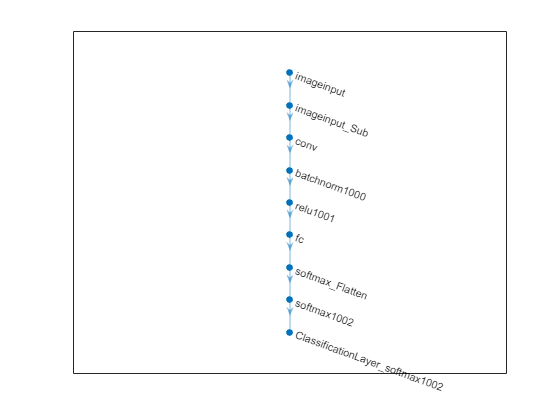Import a pretrained ONNX network as a `LayerGraph` object. Then, assemble the imported layers into a `DAGNetwork` object, and use the assembled network to classify an image.

Generate an ONNX model of the `squeezenet` convolution neural network.

```squeezeNet = squeezenet; exportONNXNetwork(squeezeNet,"squeezeNet.onnx");```

Specify the model file and the class names.

```modelfile = "squeezenet.onnx"; ClassNames = squeezeNet.Layers(end).Classes;```

Import the layers and weights of the ONNX network. By default, `importONNXLayers` imports the network as a `LayerGraph` object compatible with a `DAGNetwork` object.

`lgraph = importONNXLayers(modelfile)`
```lgraph = LayerGraph with properties: Layers: [70×1 nnet.cnn.layer.Layer] Connections: [77×2 table] InputNames: {'data'} OutputNames: {'ClassificationLayer_prob'} ```

Analyze the imported network architecture.

`analyzeNetwork(lgraph)`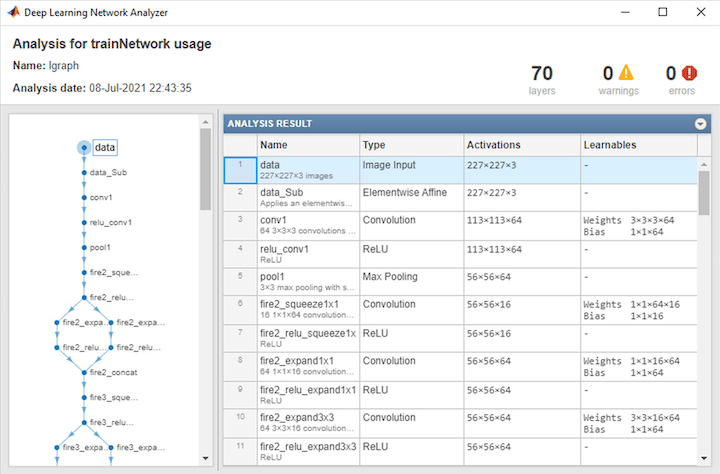Display the last layer of the imported network. The output shows that the layer graph has a `ClassificationOutputLayer` at the end of the network architecture.

`lgraph.Layers(end)`
```ans = ClassificationOutputLayer with properties: Name: 'ClassificationLayer_prob' Classes: 'auto' ClassWeights: 'none' OutputSize: 'auto' Hyperparameters LossFunction: 'crossentropyex' ```

The classification layer does not contain the classes, so you must specify these before assembling the network. If you do not specify the classes, then the software automatically sets the classes to `1`, `2`, ..., `N`, where `N` is the number of classes.

The classification layer has the name `'ClassificationLayer_prob'`. Set the classes to `ClassNames`, and then replace the imported classification layer with the new one.

```cLayer = lgraph.Layers(end); cLayer.Classes = ClassNames; lgraph = replaceLayer(lgraph,'ClassificationLayer_prob',cLayer);```

Assemble the layer graph using `assembleNetwork` to return a `DAGNetwork` object.

`net = assembleNetwork(lgraph)`
```net = DAGNetwork with properties: Layers: [70×1 nnet.cnn.layer.Layer] Connections: [77×2 table] InputNames: {'data'} OutputNames: {'ClassificationLayer_prob'} ```

Read the image you want to classify and display the size of the image. The image is 384-by-512 pixels and has three color channels (RGB).

```I = imread("peppers.png"); size(I)```
```ans = 1×3 384 512 3 ```

Resize the image to the input size of the network. Show the image.

```I = imresize(I,[227 227]); imshow(I)```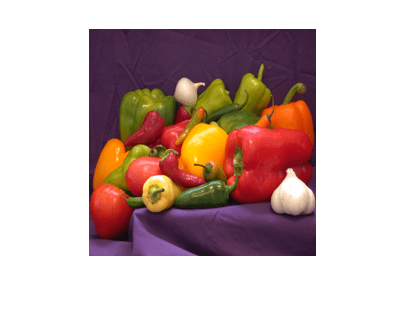Classify the image using the imported network.

`label = classify(net,I)`
```label = categorical bell pepper ```

Import a pretrained ONNX network as a `LayerGraph` object compatible with a `dlnetwork` object. Then, convert the layer graph to a `dlnetwork` to classify an image.

Generate an ONNX model of the `squeezenet` convolution neural network.

```squeezeNet = squeezenet; exportONNXNetwork(squeezeNet,"squeezeNet.onnx");```

Specify the model file and the class names.

```modelfile = "squeezenet.onnx"; ClassNames = squeezeNet.Layers(end).Classes;```

Import the layers and weights of the ONNX network. Specify to import the network as a `LayerGraph` object compatible with a `dlnetwork` object.

`lgraph = importONNXLayers(modelfile,TargetNetwork="dlnetwork")`
```lgraph = LayerGraph with properties: Layers: [70×1 nnet.cnn.layer.Layer] Connections: [77×2 table] InputNames: {'data'} OutputNames: {1×0 cell} ```

Read the image you want to classify and display the size of the image. The image is 384-by-512 pixels and has three color channels (RGB).

```I = imread("peppers.png"); size(I)```
```ans = 1×3 384 512 3 ```

Resize the image to the input size of the network. Show the image.

```I = imresize(I,[227 227]); imshow(I)```Convert the imported layer graph to a `dlnetwork` object.

`dlnet = dlnetwork(lgraph);`

Convert the image to a `dlarray`. Format the images with the dimensions `"SSCB"` (spatial, spatial, channel, batch). In this case, the batch size is 1 and you can omit it (`"SSC"`).

`I_dlarray = dlarray(single(I),"SSCB");`

Classify the sample image and find the predicted label.

```prob = predict(dlnet,I_dlarray); [~,label] = max(prob);```

Display the classification result.

`ClassNames(label)`
```ans = categorical bell pepper ```

Import a pretrained ONNX network as a `LayerGraph` object, and assemble the imported layers into a `DAGNetwork` object. Then, use the `DAGNetwork` to classify an image. The imported network contains ONNX operators that are not supported for conversion into built-in MATLAB layers. The software automatically generates custom layers when you import these operators.

This example uses the helper function `findCustomLayers`. To view the code for this function, see Helper Function.

Specify the file to import as `shufflenet` with operator set 9 from the ONNX Model Zoo. `shufflenet` is a convolutional neural network that is trained on more than a million images from the ImageNet database. As a result, the network has learned rich feature representations for a wide range of images. The network can classify images into 1000 object categories, such as keyboard, mouse, pencil, and many animals.

`modelfile = "shufflenet-9.onnx";`

Import the layers and weights of `shufflenet`. By default, `importONNXLayers` imports the network as a `LayerGraph` object compatible with a `DAGNetwork` object. If the imported network contains ONNX operators not supported for conversion into built-in MATLAB layers, then `importONNXLayers` can automatically generate custom layers in place of these layers. `importONNXLayers` saves each generated custom layer to a separate `.m` file in the package `+shufflenet_9` in the current folder. Specify the package name by using the name-value argument `PackageName`.

`lgraph = importONNXLayers(modelfile,PackageName="shufflenet_9")`
```lgraph = LayerGraph with properties: Layers: [173×1 nnet.cnn.layer.Layer] Connections: [188×2 table] InputNames: {'gpu_0_data_0'} OutputNames: {'ClassificationLayer_gpu_0_softmax_1'} ```

Find the indices of the automatically generated custom layers by using the helper function `findCustomLayers`, and display the custom layers.

```ind = findCustomLayers(lgraph.Layers,'+shufflenet_9'); lgraph.Layers(ind)```
```ans = 16×1 Layer array with layers: 1 'Reshape_To_ReshapeLayer1004' shufflenet_9.Reshape_To_ReshapeLayer1004 shufflenet_9.Reshape_To_ReshapeLayer1004 2 'Reshape_To_ReshapeLayer1009' shufflenet_9.Reshape_To_ReshapeLayer1009 shufflenet_9.Reshape_To_ReshapeLayer1009 3 'Reshape_To_ReshapeLayer1014' shufflenet_9.Reshape_To_ReshapeLayer1014 shufflenet_9.Reshape_To_ReshapeLayer1014 4 'Reshape_To_ReshapeLayer1019' shufflenet_9.Reshape_To_ReshapeLayer1019 shufflenet_9.Reshape_To_ReshapeLayer1019 5 'Reshape_To_ReshapeLayer1024' shufflenet_9.Reshape_To_ReshapeLayer1024 shufflenet_9.Reshape_To_ReshapeLayer1024 6 'Reshape_To_ReshapeLayer1029' shufflenet_9.Reshape_To_ReshapeLayer1029 shufflenet_9.Reshape_To_ReshapeLayer1029 7 'Reshape_To_ReshapeLayer1034' shufflenet_9.Reshape_To_ReshapeLayer1034 shufflenet_9.Reshape_To_ReshapeLayer1034 8 'Reshape_To_ReshapeLayer1039' shufflenet_9.Reshape_To_ReshapeLayer1039 shufflenet_9.Reshape_To_ReshapeLayer1039 9 'Reshape_To_ReshapeLayer1044' shufflenet_9.Reshape_To_ReshapeLayer1044 shufflenet_9.Reshape_To_ReshapeLayer1044 10 'Reshape_To_ReshapeLayer1049' shufflenet_9.Reshape_To_ReshapeLayer1049 shufflenet_9.Reshape_To_ReshapeLayer1049 11 'Reshape_To_ReshapeLayer1054' shufflenet_9.Reshape_To_ReshapeLayer1054 shufflenet_9.Reshape_To_ReshapeLayer1054 12 'Reshape_To_ReshapeLayer1059' shufflenet_9.Reshape_To_ReshapeLayer1059 shufflenet_9.Reshape_To_ReshapeLayer1059 13 'Reshape_To_ReshapeLayer1064' shufflenet_9.Reshape_To_ReshapeLayer1064 shufflenet_9.Reshape_To_ReshapeLayer1064 14 'Reshape_To_ReshapeLayer1069' shufflenet_9.Reshape_To_ReshapeLayer1069 shufflenet_9.Reshape_To_ReshapeLayer1069 15 'Reshape_To_ReshapeLayer1074' shufflenet_9.Reshape_To_ReshapeLayer1074 shufflenet_9.Reshape_To_ReshapeLayer1074 16 'Reshape_To_ReshapeLayer1079' shufflenet_9.Reshape_To_ReshapeLayer1079 shufflenet_9.Reshape_To_ReshapeLayer1079 ```

The classification layer does not contain the classes, so you must specify these before assembling the network. If you do not specify the classes, then the software automatically sets the classes to `1`, `2`, ..., `N`, where `N` is the number of classes.

Import the class names from `squeezenet`, which is also trained with images from the ImageNet database.

```SqueezeNet = squeezenet; classNames = SqueezeNet.Layers(end).ClassNames;```

The classification layer `cLayer` is the final layer of `lgraph`. Set the classes to `classNames` and then replace the imported classification layer with the new one.

`cLayer = lgraph.Layers(end)`
```cLayer = ClassificationOutputLayer with properties: Name: 'ClassificationLayer_gpu_0_softmax_1' Classes: 'auto' ClassWeights: 'none' OutputSize: 'auto' Hyperparameters LossFunction: 'crossentropyex' ```
```cLayer.Classes = classNames; lgraph = replaceLayer(lgraph,lgraph.Layers(end).Name,cLayer);```

Assemble the layer graph using `assembleNetwork`. The function returns a `DAGNetwork` object that is ready to use for prediction.

`net = assembleNetwork(lgraph)`
```net = DAGNetwork with properties: Layers: [173×1 nnet.cnn.layer.Layer] Connections: [188×2 table] InputNames: {'gpu_0_data_0'} OutputNames: {'ClassificationLayer_gpu_0_softmax_1'} ```

Read the image you want to classify and display the size of the image. The image is 792-by-1056 pixels and has three color channels (RGB).

```I = imread("peacock.jpg"); size(I)```
```ans = 1×3 792 1056 3 ```

Resize the image to the input size of the network. Show the image.

```I = imresize(I,[224 224]); imshow(I)```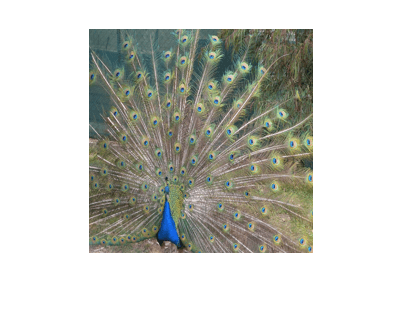The inputs to `shufflenet` require further preprocessing (for details, see ShuffleNet in ONNX Model Zoo). Rescale the image. Normalize the image by subtracting the mean of the training images and dividing by the standard deviation of the training images.

```I = rescale(I,0,1); meanIm = [0.485 0.456 0.406]; stdIm = [0.229 0.224 0.225]; I = (I - reshape(meanIm,[1 1 3]))./reshape(stdIm,[1 1 3]);```

Classify the image using the imported network.

`label = classify(net,I)`
```label = categorical peacock ```

Helper Function

This section provides the code of the helper function `findCustomLayers` used in this example. `findCustomLayers` returns the `indices` of the custom layers that `importONNXLayers` automatically generates.

```function indices = findCustomLayers(layers,PackageName) s = what(['.\' PackageName]); indices = zeros(1,length(s.m)); for i = 1:length(layers) for j = 1:length(s.m) if strcmpi(class(layers(i)),[PackageName(2:end) '.' s.m{j}(1:end-2)]) indices(j) = i; end end end end```

Import an ONNX long short-term memory (LSTM) network as a layer graph, and then find and replace the placholder layers. An LSTM network enables you to input sequence data into a network, and make predictions based on the individual time steps of the sequence data.

`lstmNet` has a similar architecture to the LSTM network created in Sequence Classification Using Deep Learning. `lstmNet` is trained to recognize the speaker given time series data representing two Japanese vowels spoken in succession.

Specify `lstmNet` as the model file.

`modelfile = "lstmNet.onnx";`

Import the layers and weights of the ONNX network. By default, `importONNXLayers` imports the network as a `LayerGraph` object compatible with a `DAGNetwork` object.

`lgraph = importONNXLayers("lstmNet.onnx")`
```Warning: Unable to import some ONNX operators, because they are not supported. They have been replaced by placeholder layers. To find these layers, call the function findPlaceholderLayers on the returned object. 1 operators(s) : Unable to create an output layer for the ONNX network output 'softmax1001' because its data format is unknown or unsupported by MATLAB output layers. If you know its format, pass it using the 'OutputDataFormats' argument. To import the ONNX network as a function, use importONNXFunction. ```
```lgraph = LayerGraph with properties: Layers: [6×1 nnet.cnn.layer.Layer] Connections: [5×2 table] InputNames: {'sequenceinput'} OutputNames: {1×0 cell} ```

`importONNXLayers` displays a warning and inserts a placeholder layer for the output layer.

You can check for placeholder layers by viewing the `Layers` property of `lgraph` or by using the `findPlaceholderLayers` function.

`lgraph.Layers`
```ans = 6×1 Layer array with layers: 1 'sequenceinput' Sequence Input Sequence input with 12 dimensions 2 'lstm1000' LSTM LSTM with 100 hidden units 3 'fc_MatMul' Fully Connected 9 fully connected layer 4 'fc_Add' Elementwise Affine Applies an elementwise scaling followed by an addition to the input. 5 'Flatten_To_SoftmaxLayer1005' lstmNet.Flatten_To_SoftmaxLayer1005 lstmNet.Flatten_To_SoftmaxLayer1005 6 'OutputLayer_softmax1001' PLACEHOLDER LAYER Placeholder for 'added_outputLayer' ONNX operator ```
`placeholderLayers = findPlaceholderLayers(lgraph)`
```placeholderLayers = PlaceholderLayer with properties: Name: 'OutputLayer_softmax1001' ONNXNode: [1×1 struct] Weights: [] Learnable Parameters No properties. State Parameters No properties. Show all properties ```

Create an output layer to replace the placeholder layer. First, create a classification layer with the name `OutputLayer_softmax1001`. If you do not specify the classes, then the software automatically sets them to `1`, `2`, ..., `N`, where `N` is the number of classes. In this case, the class data is a categorical vector of labels "1","2",..."9", which correspond to nine speakers.

`outputLayer = classificationLayer('Name','OutputLayer_softmax1001');`

Replace the placeholder layers with `outputLayer` by using the `replaceLayer` function.

`lgraph = replaceLayer(lgraph,'OutputLayer_softmax1001',outputLayer);`

Display the `Layers` property of the layer graph to confirm the replacement.

`lgraph.Layers`
```ans = 6×1 Layer array with layers: 1 'sequenceinput' Sequence Input Sequence input with 12 dimensions 2 'lstm1000' LSTM LSTM with 100 hidden units 3 'fc_MatMul' Fully Connected 9 fully connected layer 4 'fc_Add' Elementwise Affine Applies an elementwise scaling followed by an addition to the input. 5 'Flatten_To_SoftmaxLayer1005' lstmNet.Flatten_To_SoftmaxLayer1005 lstmNet.Flatten_To_SoftmaxLayer1005 6 'OutputLayer_softmax1001' Classification Output crossentropyex ```

Alternatively, define the output layer when you import the layer graph by using the `OutputLayerType` or `OutputDataFormats` option. Check if the imported layer graphs have placeholder layers by using `findPlaceholderLayers`.

```lgraph1 = importONNXLayers("lstmNet.onnx",OutputLayerType="classification"); findPlaceholderLayers(lgraph1)```
```ans = 0×1 Layer array with properties: ```
```lgraph2 = importONNXLayers("lstmNet.onnx",OutputDataFormats="BC"); findPlaceholderLayers(lgraph2)```
```ans = 0×1 Layer array with properties: ```

The imported layer graphs `lgraph1` and `lgraph2` do not have placeholder layers.

Import an ONNX network that has multiple outputs by using `importONNXLayers`, and then assemble the imported layer graph into a `DAGNetwork` object.

Specify the network file from which to import layers and weights.

`modelfile = "digitsMIMO.onnx";`

Import the layers and weights from `modelfile`. The network in `digitsMIMO.onnx` has two output layers: one classification layer (`ClassificationLayer_sm_1`) to classify digits and one regression layer (`RegressionLayer_fc_1_Flatten`) to compute the mean squared error for the predicted angles of the digits.

`lgraph = importONNXLayers(modelfile)`
```lgraph = LayerGraph with properties: Layers: [19×1 nnet.cnn.layer.Layer] Connections: [19×2 table] InputNames: {'input'} OutputNames: {'ClassificationLayer_sm_1' 'RegressionLayer_fc_1_Flatten'} ```

Plot the layer graph using `plot`, and display the layers of `lgraph`.

`plot(lgraph)`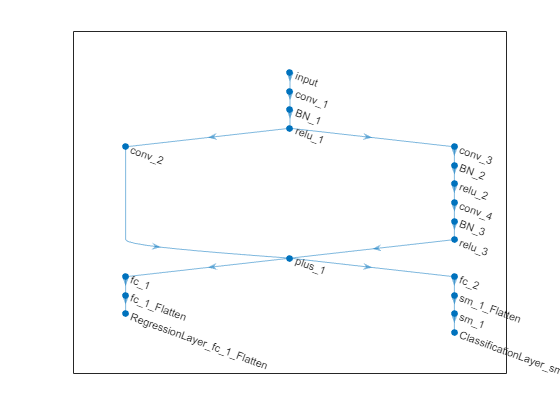`lgraph.Layers`
```ans = 19×1 Layer array with layers: 1 'input' Image Input 28×28×1 images 2 'conv_1' Convolution 16 5×5×1 convolutions with stride [1 1] and padding [2 2 2 2] 3 'BN_1' Batch Normalization Batch normalization with 16 channels 4 'relu_1' ReLU ReLU 5 'conv_2' Convolution 32 1×1×16 convolutions with stride [2 2] and padding [0 0 0 0] 6 'conv_3' Convolution 32 3×3×16 convolutions with stride [2 2] and padding [1 1 1 1] 7 'BN_2' Batch Normalization Batch normalization with 32 channels 8 'relu_2' ReLU ReLU 9 'conv_4' Convolution 32 3×3×32 convolutions with stride [1 1] and padding [1 1 1 1] 10 'BN_3' Batch Normalization Batch normalization with 32 channels 11 'relu_3' ReLU ReLU 12 'plus_1' Addition Element-wise addition of 2 inputs 13 'fc_1' Convolution 1 14×14×32 convolutions with stride [1 1] and padding [0 0 0 0] 14 'fc_2' Convolution 10 14×14×32 convolutions with stride [1 1] and padding [0 0 0 0] 15 'sm_1_Flatten' ONNX Flatten Flatten activations into 1-D assuming C-style (row-major) order 16 'sm_1' Softmax softmax 17 'fc_1_Flatten' ONNX Flatten Flatten activations into 1-D assuming C-style (row-major) order 18 'ClassificationLayer_sm_1' Classification Output crossentropyex 19 'RegressionLayer_fc_1_Flatten' Regression Output mean-squared-error ```

The classification layer does not contain the classes, so you must specify these before assembling the network. If you do not specify the classes, then the software automatically sets the classes to `1`, `2`, ..., `N`, where `N` is the number of classes. Specify the classes of `cLayer` as `0`, `1`, ..., `9.` Then, replace the imported classification layer with the new one.

```ClassNames = string(0:9); cLayer = lgraph.Layers(18); cLayer.Classes = ClassNames; lgraph = replaceLayer(lgraph,"ClassificationLayer_sm_1",cLayer);```

Assemble the layer graph using `assembleNetwork`. The function returns a `DAGNetwork` object that is ready to use for prediction.

`assembledNet = assembleNetwork(lgraph)`
```assembledNet = DAGNetwork with properties: Layers: [19×1 nnet.cnn.layer.Layer] Connections: [19×2 table] InputNames: {'input'} OutputNames: {'ClassificationLayer_sm_1' 'RegressionLayer_fc_1_Flatten'} ```

## Input Arguments

collapse all

Name of the ONNX model file containing the network, specified as a character vector or string scalar. The file must be in the current folder or in a folder on the MATLAB path, or you must include a full or relative path to the file.

Example: `"cifarResNet.onnx"`

### Name-Value Arguments

Specify optional pairs of arguments as `Name1=Value1,...,NameN=ValueN`, where `Name` is the argument name and `Value` is the corresponding value. Name-value arguments must appear after other arguments, but the order of the pairs does not matter.

Example: `importONNXLayers(modelfile,TargetNetwork="dagnetwork",GenerateCustomLayers=true,PackageName="CustomLayers")` imports the network layers from `modelfile` as a layer graph compatible with a `DAGNetwork` object and saves the automatically generated custom layers in the package `+CustomLayers` in the current folder.

Option for custom layer generation, specified as a numeric or logical `1` (`true`) or `0` (`false`). If you set `GenerateCustomLayers` to `true`, `importONNXLayers` tries to generate a custom layer when the software cannot convert an ONNX operator into an equivalent built-in MATLAB layer. `importONNXLayers` saves each generated custom layer to a separate `.m` file in `+PackageName`. To view or edit a custom layer, open the associated `.m` file. For more information on custom layers, see Deep Learning Custom Layers.

Example: `GenerateCustomLayers=false`

Name of the package in which `importONNXLayers` saves custom layers, specified as a character vector or string scalar. `importONNXLayers` saves the custom layers package `+PackageName` in the current folder. If you do not specify `PackageName`, then `importONNXLayers` saves the custom layers in a package named `+modelfile` in the current folder. For more information on packages, see Packages Create Namespaces.

Example: `PackageName="shufflenet_9"`

Example: `PackageName="CustomLayers"`

Target type of Deep Learning Toolbox network for imported network architecture, specified as `"dagnetwork"` or `"dlnetwork"`. The function `importONNXLayers` imports the network architecture as a `LayerGraph` object compatible with a `DAGNetwork` or `dlnetwork` object.

• If you specify `TargetNetwork` as `"dagnetwork"`, the imported `lgraph` must include input and output layers specified by the ONNX model or that you specify using the name-value arguments `InputDataFormats`, `OutputDataFormats`, or `OutputLayerType`.

• If you specify `TargetNetwork` as `"dlnetwork"`, `importONNXLayers` appends a `CustomOutputLayer` at the end of each output branch of `lgraph`, and might append a `CustomInputLayer` at the beginning of an input branch. The function appends a `CustomInputLayer` if the input data formats or input image sizes are not known. For network-specific information on the data formats of these layers, see the properties of the `CustomInputLayer` and `CustomOutputLayer` objects. For information on how to interpret Deep Learning Toolbox input and output data formats, see Conversion of ONNX Input and Output Tensors into Built-In MATLAB Layers.

Example: `TargetNetwork="dlnetwork"` imports a `LayerGraph` object compatible with a `dlnetwork` object.

Data format of the network inputs, specified as a character vector, string scalar, or string array. `importONNXLayers` tries to interpret the input data formats from the ONNX file. The name-value argument `InputDataFormats` is useful when `importONNXLayers` cannot derive the input data formats.

Set `InputDataFomats` to a data format in the ordering of an ONNX input tensor. For example, if you specify `InputDataFormats` as `"BSSC"`, the imported network has one `imageInputLayer` input. For more information on how `importONNXLayers` interprets the data format of ONNX input tensors and how to specify `InputDataFormats` for different Deep Learning Toolbox input layers, see Conversion of ONNX Input and Output Tensors into Built-In MATLAB Layers.

If you specify an empty data format (`[]` or `""`), `importONNXLayers` automatically interprets the input data format.

Example: `InputDataFormats='BSSC'`

Example: `InputDataFormats="BSSC"`

Example: `InputDataFormats=["BCSS","","BC"]`

Example: `InputDataFormats={'BCSS',[],'BC'}`

Data Types: `char` | `string` | `cell`

Data format of the network outputs, specified as a character vector, string scalar, or string array. `importONNXLayers` tries to interpret the output data formats from the ONNX file. The name-value argument `OutputDataFormats` is useful when `importONNXLayers` cannot derive the output data formats.

Set `OutputDataFormats` to a data format in the ordering of an ONNX output tensor. For example, if you specify `OutputDataFormats` as `"BC"`, the imported network has one `classificationLayer` output. For more information on how `importONNXLayers` interprets the data format of ONNX output tensors and how to specify `OutputDataFormats` for different Deep Learning Toolbox output layers, see Conversion of ONNX Input and Output Tensors into Built-In MATLAB Layers.

If you specify an empty data format (`[]` or `""`), `importONNXLayers` automatically interprets the output data format.

Example: `OutputDataFormats='BC'`

Example: `OutputDataFormats="BC"`

Example: `OutputDataFormats=["BCSS","","BC"]`

Example: `OutputDataFormats={'BCSS',[],'BC'}`

Data Types: `char` | `string` | `cell`

Size of the input image for the first network input, specified as a vector of three or four numerical values corresponding to `[height,width,channels]` for 2-D images and `[height,width,depth,channels]` for 3-D images. The network uses this information only when the ONNX model in `modelfile` does not specify the input size.

Example: `ImageInputSize=[28 28 1]` for a 2-D grayscale input image

Example: `ImageInputSize=[224 224 3]` for a 2-D color input image

Example: `ImageInputSize=[28 28 36 3]` for a 3-D color input image

Layer type for the first network output, specified as `"classification"`, `"regression"`, or `"pixelclassification"`. The function `importONNXLayers` appends a `ClassificationOutputLayer`, `RegressionOutputLayer`, or `pixelClassificationLayer` (Computer Vision Toolbox) object to the end of the first output branch of the imported network architecture. Appending a `pixelClassificationLayer` (Computer Vision Toolbox) object requires Computer Vision Toolbox™. If the ONNX model in `modelfile` specifies the output layer type or you specify `TargetNetwork` as `"dlnetwork"`, `importONNXLayers` ignores the name-value argument `OutputLayerType`.

Example: `OutputLayerType="regression"`

Constant folding optimization, specified as `"deep"`, `"shallow"`, or `"none"`. Constant folding optimizes the imported network architecture by computing operations on ONNX initializers (initial constant values) during the conversion of ONNX operators to equivalent built-in MATLAB layers.

If the ONNX network contains operators that the software cannot convert to equivalent built-in MATLAB layers (see ONNX Operators Supported for Conversion into Built-In MATLAB Layers), then `importONNXLayers` inserts a placeholder layer in place of each unsupported layer. For more information, see Tips.

Constant folding optimization can reduce the number of placeholder layers. When you set `FoldConstants` to `"deep"`, the imported layers include the same or fewer placeholder layers, compared to when you set the argument to `"shallow"`. However, the importing time might increase. Set `FoldConstants` to `"none"` to disable the network architecture optimization.

Example: `FoldConstants="shallow"`

## Output Arguments

collapse all

Network architecture of the pretrained ONNX model, returned as a `LayerGraph` object.

To use the imported layer graph for prediction, you must convert the `LayerGraph` object to a `DAGNetwork` or `dlnetwork` object. Specify the name-value argument `TargetNetwork` as `"dagnetwork"` or `"dlnetwork"` depending on the intended workflow.

## Limitations

• `importONNXLayers` supports ONNX versions as follows:

• The function supports ONNX intermediate representation version 6.

• The function supports ONNX operator sets 6 to 13.

Note

If you import an exported network, layers of the reimported network might differ from the original network and might not be supported.

collapse all

### ONNX Operators Supported for Conversion into Built-In MATLAB Layers

`importONNXLayers` supports the following ONNX operators for conversion into built-in MATLAB layers, with some limitations.

ONNX OperatorDeep Learning Toolbox Layer

`Add`

`additionLayer` or `nnet.onnx.layer.ElementwiseAffineLayer`

`AveragePool`

`averagePooling2dLayer`

`BatchNormalization`

`batchNormalizationLayer`

`Concat`

`concatenationLayer`

`Constant`

None (Imported as weights)

`Conv`*

`convolution2dLayer`

`ConvTranspose`

`transposedConv2dLayer`

`Dropout`

`dropoutLayer`

`Elu`

`eluLayer`

`Gemm`

`fullyConnectedLayer` if ONNX network is recurrent, otherwise `nnet.onnx.layer.FlattenLayer` followed by `convolution2dLayer`

`GlobalAveragePool`

`globalAveragePooling2dLayer`

`GlobalMaxPool`

`globalMaxPooling2dLayer`

`GRU`

`gruLayer`

`InstanceNormalization`

`groupNormalizationLayer` with `numGroups` specified as `"channel-wise"`

`LeakyRelu`

`leakyReluLayer`

`LRN`

`CrossChannelNormalizationLayer`

`LSTM`

`lstmLayer` or `bilstmLayer`

`MatMul`

`fullyConnectedLayer` if ONNX network is recurrent, otherwise `convolution2dLayer`

`MaxPool`

`maxPooling2dLayer`

`Mul`

`multiplicationLayer`

`Relu`

`reluLayer` or `clippedReluLayer`

`Sigmoid`

`sigmoidLayer`

`Softmax`

`softmaxLayer`

`Sum`

`additionLayer`

`Tanh`

`tanhLayer`

*If the `pads` attribute of the `Conv` operator is a vector with only two elements `[p1,p2]`, `importONNXLayers` imports `Conv` as a `convolution2dLayer` with the name-value argument `'Padding'` specified as `[p1,p2,p1,p2]`.

ONNX OperatorONNX Importer Custom Layer

`Clip`

`nnet.onnx.layer.ClipLayer`

`Div`

`nnet.onnx.layer.ElementwiseAffineLayer`

`Flatten`

`nnet.onnx.layer.FlattenLayer` or `nnet.onnx.layer.Flatten3dLayer`

`Identity`

`nnet.onnx.layer.IdentityLayer`

`ImageScaler`

`nnet.onnx.layer.ElementwiseAffineLayer`

`PRelu`

`nnet.onnx.layer.PReluLayer`

`Reshape`

`nnet.onnx.layer.FlattenLayer`

`Sub`

`nnet.onnx.layer.ElementwiseAffineLayer`
ONNX OperatorImage Processing Toolbox™
`DepthToSpace``depthToSpace2dLayer` (Image Processing Toolbox)
`Resize``resize2dLayer` (Image Processing Toolbox) or `resize3dLayer` (Image Processing Toolbox)
`SpaceToDepth``spaceToDepthLayer` (Image Processing Toolbox)
`Upsample``resize2dLayer` (Image Processing Toolbox) or `resize3dLayer` (Image Processing Toolbox)

### Conversion of ONNX Input and Output Tensors into Built-In MATLAB Layers

`importONNXLayers` tries to interpret the data format of the ONNX network's input and output tensors, and then convert them into built-in MATLAB input and output layers. For details on the interpretation, see the tables Conversion of ONNX Input Tensors into Deep Learning Toolbox Layers and Conversion of ONNX Output Tensors into MATLAB Layers.

In Deep Learning Toolbox, each data format character must be one of these labels:

• `S` — Spatial

• `C` — Channel

• `B` — Batch observations

• `T` — Time or sequence

• `U` — Unspecified

Conversion of ONNX Input Tensors into Deep Learning Toolbox Layers

Data FormatsData InterpretationDeep Learning Toolbox Layer
ONNX Input Tensor MATLAB Input FormatShapeType
`BC``CB`c-by-n array, where c is the number of features and n is the number of observationsFeatures`featureInputLayer`
`BCSS`, `BSSC`, `CSS`, `SSC``SSCB`

h-by-w-by-c-by-n numeric array, where h, w, c and n are the height, width, number of channels of the images, and number of observations, respectively

2-D image`imageInputLayer`
`BCSSS`, `BSSSC`, `CSSS`, `SSSC``SSSCB`

h-by-w-by-d-by-c numeric array, where h, w, d, c and n are the height, width, depth, number of channels of the images, and number of image observations, respectively

3-D image`image3dInputLayer`
`TBC``CBT`

c-by-s-by-n matrix, where c is the number of features of the sequence, s is the sequence length, and n is the number of sequence observations

Vector sequence`sequenceInputLayer`
`TBCSS``SSCBT`

h-by-w-by-c-by-s-by-n array, where h, w, c and n correspond to the height, width, and number of channels of the image, respectively, s is the sequence length, and n is the number of image sequence observations

2-D image sequence`sequenceInputLayer`
`TBCSSS``SSSCBT`

h-by-w-by-d-by-c-by-s-by-n array, where h, w, d, and c correspond to the height, width, depth, and number of channels of the image, respectively, s is the sequence length, and n is the number of image sequence observations

3-D image sequence`sequenceInputLayer`

Conversion of ONNX Output Tensors into MATLAB Layers

Data FormatsMATLAB Layer
ONNX Output TensorMATLAB Output Format
`BC`, `TBC``CB`, `CBT``classificationLayer`
`BCSS`, `BSSC`, `CSS`, `SSC`, `BCSSS`, `BSSSC`, `CSSS`, `SSSC``SSCB`, `SSSCB``pixelClassificationLayer` (Computer Vision Toolbox)
`TBCSS`, `TBCSSS``SSCBT`, `SSSCBT``regressionLayer`

### Use Imported Network Layers on GPU

`importONNXLayers` does not execute on a GPU. However, `importONNXLayers` imports the layers of a pretrained neural network for deep learning as a `LayerGraph` object, which you can use on a GPU.

• Convert the imported `LayerGraph` object to a `DAGNetwork` object by using `assembleNetwork`. On the `DAGNetwork` object, you can then predict class labels on either a CPU or GPU by using `classify`. Specify the hardware requirements using the name-value argument `ExecutionEnvironment`. For networks with multiple outputs, use the `predict` function and specify the name-value argument `ReturnCategorical` as `true`.

• Convert the imported `LayerGraph` object to a `dlnetwork` object by using `dlnetwork`. On the `dlnetwork` object, you can then predict class labels on either a CPU or GPU by using `predict`. The function `predict` executes on the GPU if either the input data or network parameters are stored on the GPU.

• If you use `minibatchqueue` to process and manage the mini-batches of input data, the `minibatchqueue` object converts the output to a GPU array by default if a GPU is available.

• Use `dlupdate` to convert the learnable parameters of a `dlnetwork` object to GPU arrays.

`dlnet = dlupdate(@gpuarray,dlnet)`

• You can train the imported `LayerGraph` object on either a CPU or GPU by using `trainNetwork`. To specify training options, including options for the execution environment, use the `trainingOptions` function. Specify the hardware requirements using the name-value argument `ExecutionEnvironment`. For more information on how to accelerate training, see Scale Up Deep Learning in Parallel, on GPUs, and in the Cloud.

Using a GPU requires Parallel Computing Toolbox™ and a supported GPU device. For information on supported devices, see GPU Support by Release (Parallel Computing Toolbox).

## Tips

• If the imported network contains an ONNX operator not supported for conversion into a built-in MATLAB layer (see ONNX Operators Supported for Conversion into Built-In MATLAB Layers) and `importONNXLayers` does not generate a custom layer, then `importONNXLayers` inserts a placeholder layer in place of the unsupported layer. To find the names and indices of the unsupported layers in the network, use the `findPlaceholderLayers` function. You then can replace a placeholder layer with a new layer that you define. To replace a layer, use `replaceLayer`. For an example, see Import and Assemble ONNX Network with Multiple Outputs.

• To use a pretrained network for prediction or transfer learning on new images, you must preprocess your images in the same way the images that were used to train the imported model were preprocessed. The most common preprocessing steps are resizing images, subtracting image average values, and converting the images from BGR images to RGB.

• To resize images, use `imresize`. For example, `imresize(image,[227,227,3])`.

• To convert images from RGB to BGR format, use `flip`. For example, `flip(image,3)`.

For more information on preprocessing images for training and prediction, see Preprocess Images for Deep Learning.

## Alternative Functionality

Deep Learning Toolbox Converter for ONNX Model Format provides three functions to import a pretrained ONNX network: `importONNXNetwork`, `importONNXLayers`, and `importONNXFunction`. For more information on which import function best suits different scenarios, see Select Function to Import ONNX Pretrained Network.

## Compatibility Considerations

expand all

Warns starting in R2021b

Behavior changed in R2021b

 Open Neural Network Exchange. https://github.com/onnx/.

 ONNX. https://onnx.ai/.